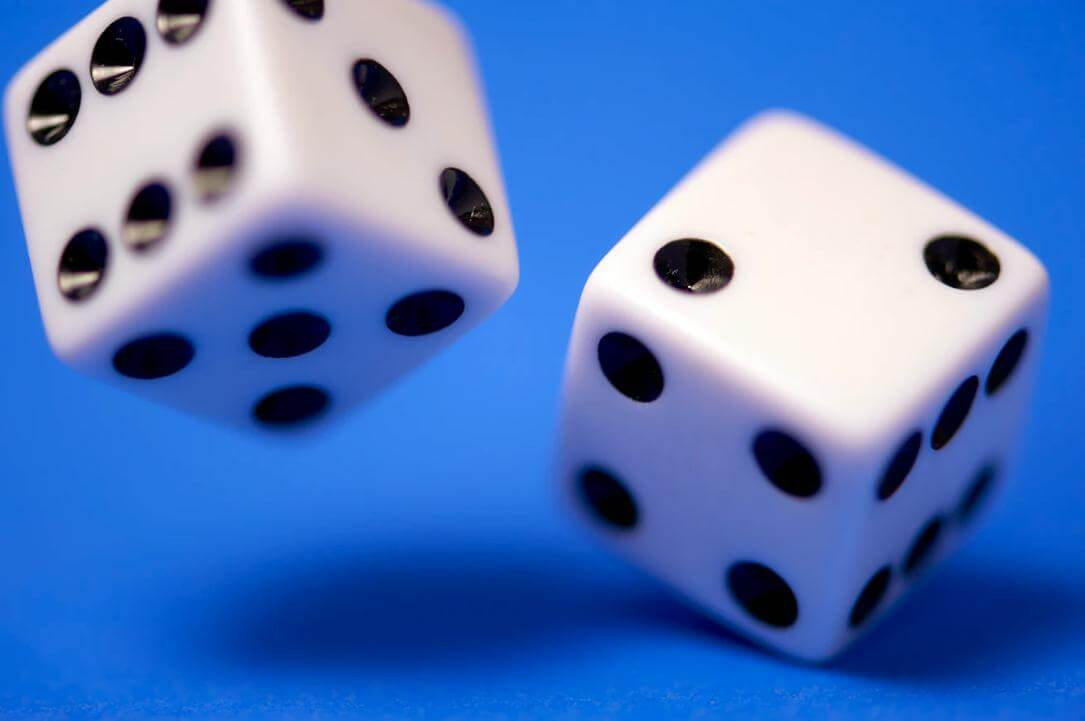This is a nice and easy coding exercise for beginners.We can use this code snippet to emulate a dice. We’ll use `Math.random()`, which returns a value between `0` and `0.99`.

All we need to do now, to make this work, is to multiply our random number with `6`.

Let’s say we got `0.99` from our `Math.random()` call; after multiplying it by `6`, the number we’ll get is `5.94`. So we need to round this number. We could round it to the closest integer with `Math.round()`, so:

If the result was `0.99` again, it would get rounded to `6`. Great! But what if the result was `0.01`?

Here’s some code to quickly test what would happen:

This would return `0`, but that’s obviously not what we want; we want a range between `1` and `6`. We can try using `Math.ceil()` (as in “ceiling”), so that if we get `0.01` as the output of `Math.random()`, the end result would be `1`. Let’s run our quick test again to confirm this:

Indeed, we get back a one. However, we’re not fully done with our dice emulator. This is due to the fact that the range of results of `Math.random()` is from zero (inclusive) to one (exclusive). In other words, when we ran `Math.random()`, we can get back `0`, `0.99`, or any number in between. This means that in the rare case of us getting a zero, our `Math.ceil()` approach will not work any more, because the `Math.ceil(0)` returs a zero.

The solution to the above issue is simple. Instead of `Math.ceil()`, we’ll use `Math.floor()`, but we’ll add a `+1` to our code. That way, if we get a zero, it’s already floored, so we’ll just add a `+1`. However, if we get, say, a `0.01`, we’ll floor it to zero, then add a `+1` to get `1`.

Here’s the updated code:

The above code will work. However, to make sure that it indeed does work, we can run a test, in which we’ll replace the `Math.random()` bit above with a few values: `0`, `0.01`, and `0.99`.

Let’s first run a quick test for a zero:

Next, let’s test `0.01`:

Finally, let’s test `0.99`:

That’s it, we’ve successfully written code that can emulate a throw of a six-sided dice.

Note:
This exercise comes from Book 3 of my book series on JS, available on Leanpub.com.